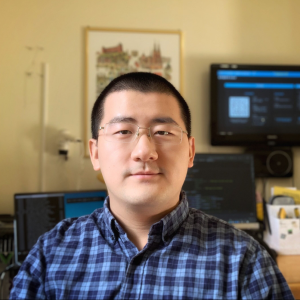# Natural Computation Methods for Machine Learning Note 15

2020年3月28日 3801点热度 1人点赞 0条评论

# Natural Computation Methods for Machine Learning Note 15

In last post, we talked Computational tools, including

• Cellular automata
• Ant Colony Optimization
• Particle Swarm Optimization

Particle Swarm Optimization

## Particle Swarm Optimization

• Originally intended to simulate bird flocks and to model social interaction (but stands on its own as an optimization tool)
• A population of particles
• Population sizes, typically 10-50 (smaller than in EC)
• A particle, i, has a position, x_i, and a velocity, v_i (Both vectors in n-dimensional space)
• Each particle’s position, x_i, represents one solution to the problem.
• Each particle remembers the best position it has found, so far, p_i

## The flying particle

• The particle "fly" through n-dimension space, in search for the best solution
• $x_{i, d}(t)=x_{i, d}(t-1)+v_{i, d}(t)$
• The velocities, v, depend on previous experience of this particle and that of its neighbors Discrete time => velocity => step length
• Neighbourhood definition varies

• Extreme cases: pbest (personal) and gbest (global)
• General case: lbest (local best)
• pbest and gbest are special cases

### Personal best (pbest)

No interaction between particles

For all particles, i, and all dimensions, d:

v_{i, d}(t)=v_{i, d}(t-1)+\mathrm{U}(0, \varphi) *\left(p_{i, d}-x_{i, d}(t-1)\right)

• U Uniformly distributed random number.
• $\varphi$ acceleration constant. (typically ≈ 2)
• $p_{i, d}$ best position found so far by particle i

### Global best (gbest)

Global interaction

For all particles, i, and all dimensions, d:

v_{i, d}(t)=v_{i, d}(t-1)+\mathrm{U}\left(0, \varphi_{1}\right) * \left(p_{i, d}-x_{i, d}(t-1)\right)+\mathrm{U}\left(0, \varphi_{2}\right) * \left(p_{g, d}-x_{i, d}(t-1)\right)

U_1+U_2

Cognitive component + Social component

• $p_{g, d}$ Best solution found so far by any particle

### Local best (lbest)

Local interaction

For all particles, i, and all dimensions, d:

$v_{i, d}(t)=v_{i, d}(t-1)+\mathrm{U}(0, \varphi_{1})(p_{i, d}-x_{i, d}(t-1))+\mathrm{U}(0, \varphi_{2})(p_{l, d}-x_{i, d}(t-1))$

• $p_{l, d}$ Best solution found so far by any particle among i’s neighbours (in some structure)

Nice, local, realistic, slower than gbest. Less risk of premature convergence

Note:

• Usually requires a speed limit (V_{max})
• Actual velocity (v) usually close to V_{max}
• Discrete time => velocity = step length
• Low accuracy close to global optimum
• Decaying Vmax?
• Imposes a time limit to reach the goal

### Constriction

• Constrict swarm with a factor K
• to avoid divergence (”explosion”)
• no longer need a speed limit

• lowers speed around global optimum

$v_{i, d}(t)=K * v_{i, d} (t-1)+U(0, \varphi_{1})(p_{i, d}-x_{i, d}(t-1))+U(0, \varphi_{2})(p_{l, d}-x_{i, d}(t-1))K=\frac{2}{\varphi-2+\sqrt{\varphi^{2}-4 \varphi}},where\varphi=\varphi_{1}+\varphi_{2}>4$

• K is a function of \varphi_{1} and \varphi_{2} only => K is constant

• yet it gives the swarm a converging behaviour, as if K was a decaying variable

### Binary particle swarms

• Velocities updated as before
• The positions:

x_{i, d}= \begin{cases} 1,&\text{if } U(0,1)

\text { logistic }\left(v_{i d}\right)=\frac{1}{1+e^{-v_{i, d}}}

• $V_{\max }=\pm 4$

• in order not to saturate the sigmoid
• so that there is at least some probability. (0.018) of a bit flipping

### What makes PSO special?

• Its simplicity